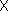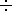#Summary of Rules### The following rules concern operations with fractions. Click on the rule you wish to review.

#### Rule 1: Zero in the Denominator of a Fraction Rule 2: Zero in the Numerator of a Fraction Rule 3: One Minus Sign in a Fraction Rule 4: Odd Number and Even Number of Minus Signs in a Fraction Rule 5: Division Symbol in a Fraction Rule 6: Properties of the Number 1 Rule 7: Different Faces of the Number 1 Rule 8: Any Integer can be Written as a Fraction Rule 9: Factoring Integers Rule 10: Reducing Fractions Rule 11: Multiplying Two Simple Fractions Rule 12: Multiplying an Integer and a Simple Fraction Rule 13: Multiplying Three or More Simple Fractions Rule 14: Dividing Simple Fractions Rule 15: Dividing a Simple Fraction by an Integer Rule 16: Dividing Three or More Simple Fractions Rule 17: Building Fractions Rule 18: Adding Two Simple Fractions Rule 19: Subtracting Two Simple Fractions Rule 20: Order of Operations for,, +, - Rule 21: Order of Operations for Parentheses Rule 22: Order of Operations for Nested Parentheses Rule 23: Order of Operations in Fractions Rule 24: Converting Complex Fractions to Simple Fractions Rule 25: Multiplying and Dividing Two Complex Fractions Rule 26: Adding and Subtracting Two Complex Fractions Rule 27: Compound Fractions Rule 28: Converting Decimals to Fractions Rule 29: Converting Percentages to Fractions[Simple Fractions] [Complex Fractions] [Compound Fractions]
[Decimals] [Percentage] [Rules]

[Algebra] [Trigonometry] [Complex Variables]S.O.S MATHematics home page

Do you need more help? Please post your question on our S.O.S. Mathematics CyberBoard.Author: Nancy Marcus